QuestionAnswers

# Construct a triangle of sides 4 cm, 5 cm and 6 cm and then a triangle similar to it whose sides are (3/5) time of the corresponding sides of the given triangle.Hint: In this particular construction problem always have a basic gist of the ruler and compass and always construct the given construction step by step, so first draw the given triangle then draw the similar triangle. use the concepts of similar triangles to reach the solution of the question.

Given data:
Triangle having sides 4 cm, 5 cm and 6 cm.
Another similar triangle whose sides are (3/5) time of the corresponding sides of the given triangle.
Steps of construction:
$\left( i \right)$ Draw a horizontal line BC of 4 cm using a ruler.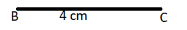$\left( {ii} \right)$ Now take a compass with pointed end at B and make an arc with pencil end at 5 cm above line BC as shown in the below figure.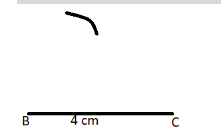$\left( {iii} \right)$ Now again take a compass with pointed end at C and make an arc with pencil end at 6 cm above line BC, which cut the previous arc at point A as shown in the below figure, now join AB and AC.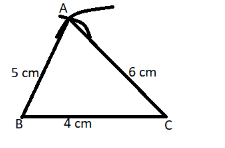So this is the required triangle of sides 4 cm, 5 cm and 6 cm.
$\left( {iv} \right)$ Now make a line parallel to line AC from point B downwards as shown in the below figure, and on this line mark five points at interval of 1 cm as shown in the figure below.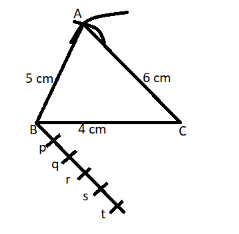$\left( v \right)$ Now from point (r) as shown in the above figure draw a parallel line to AB which cut the line BC at point C’ and join them as shown in the figure.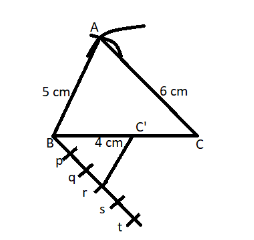$\left( {vi} \right)$ Now from point C’ make a parallel line to AC which cuts the line AB at point A’ and join them as shown in the below figure.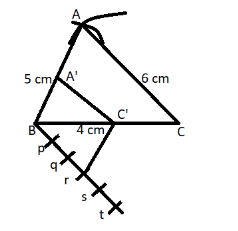So A’BC’ is the required similar triangle whose sides are (3/5) time of the corresponding sides of the given triangle.
So this is the required triangle.

Note: Whenever we face such types of questions the key concept we have to remember is that always recall all the steps which is written above these steps are the basis of the construction without these steps we cannot draw the similar triangle, so by using these steps triangle A’BC’ is the required similar triangle whose sides are (3/5) time of the corresponding sides of the given triangle.
CBSE Class 7 Maths Chapter 6 - Triangle and Its Properties FormulasCBSE Class 8 Maths Chapter 6 - Squares and Square Roots FormulasCBSE Class 8 Maths Chapter 12 - Exponents and Powers FormulasArea of Triangle FormulaCBSE Class 8 Maths Chapter 9 - Algebraic Expressions and Identities FormulasCBSE Class 8 Maths Chapter 7 - Cubes and Cube Roots FormulasCBSE Class 8 Maths Chapter 13 - Direct and Inverse Proportions FormulasCBSE Class 6 Maths Chapter 12 - Ratio and Proportion FormulasCBSE Class 8 Maths Chapter 4 - Practical Geometry FormulasCBSE Class 8 Maths Chapter 5 - Data Handling FormulasImportant Questions for CBSE Class 8 Social Science Resources and Development Chapter 5 - IndustriesImportant Questions for CBSE Class 8 Social Science Resources and Development Chapter 4 - AgricultureImportant Questions for CBSE Class 8 Social Science - Social and Political Life Chapter 4 - Understanding LawsImportant Questions for CBSE Class 8 Social Science - Social and Political Life Chapter 5 - JudiciaryImportant Questions for CBSE Class 8 Social Science Resources and Development Chapter 6 - Human ResourcesImportant Questions for CBSE Class 8 Social Science - Social and Political Life Chapter 6 - Understanding Our Criminal Justice SystemImportant Questions for CBSE Class 6 Social Science - Social and Political Life Chapter 8 - Rural LivelihoodsImportant Questions for CBSE Class 6 Social Science - Social and Political Life Chapter 4 - Key Elements Of Democratic GovernmentImportant Questions for CBSE Class 8 Maths Chapter 5 - Data HandlingImportant Questions for CBSE Class 8 Maths Chapter 4 - Practical GeometryCBSE Class 10 Hindi A Question Paper 2020Hindi A Class 10 CBSE Question Paper 2009Hindi A Class 10 CBSE Question Paper 2015Hindi A Class 10 CBSE Question Paper 2016Hindi A Class 10 CBSE Question Paper 2012Hindi A Class 10 CBSE Question Paper 2010Hindi A Class 10 CBSE Question Paper 2007Hindi A Class 10 CBSE Question Paper 2013Hindi A Class 10 CBSE Question Paper 2008Hindi A Class 10 CBSE Question Paper 2014RS Aggarwal Solutions Class 8 Chapter-5 Playing with Numbers (Ex 5D) Exercise 5.4RS Aggarwal Solutions Class 6 Chapter-8 Algebraic Expressions (Ex 8D) Exercise 8.4RD Sharma Class 6 Solutions Chapter 4 - Operations on Whole Numbers (Ex 4.5) Exercise 4.5RS Aggarwal Solutions Class 8 Chapter-6 Operations on Algebraic Expressions (Ex 6D) Exercise 6.4RD Sharma Class 8 Solutions Chapter 6 - Algebraic Expressions and Identities (Ex 6.4) Exercise 6.4RD Sharma Class 8 Solutions Chapter 6 - Algebraic Expressions and Identities (Ex 6.5) Exercise 6.5RD Sharma Class 6 Solutions Chapter 5 - Negative Numbers and Integers (Ex 5.4) Exercise 5.4RS Aggarwal Solutions Class 6 Chapter-5 Fractions (Ex 5D) Exercise 5.4RS Aggarwal Solutions Class 6 Chapter-4 Integers (Ex 4E) Exercise 4.5NCERT Solutions Class 8 Maths Maths Chapter 4 Exercise 4.5